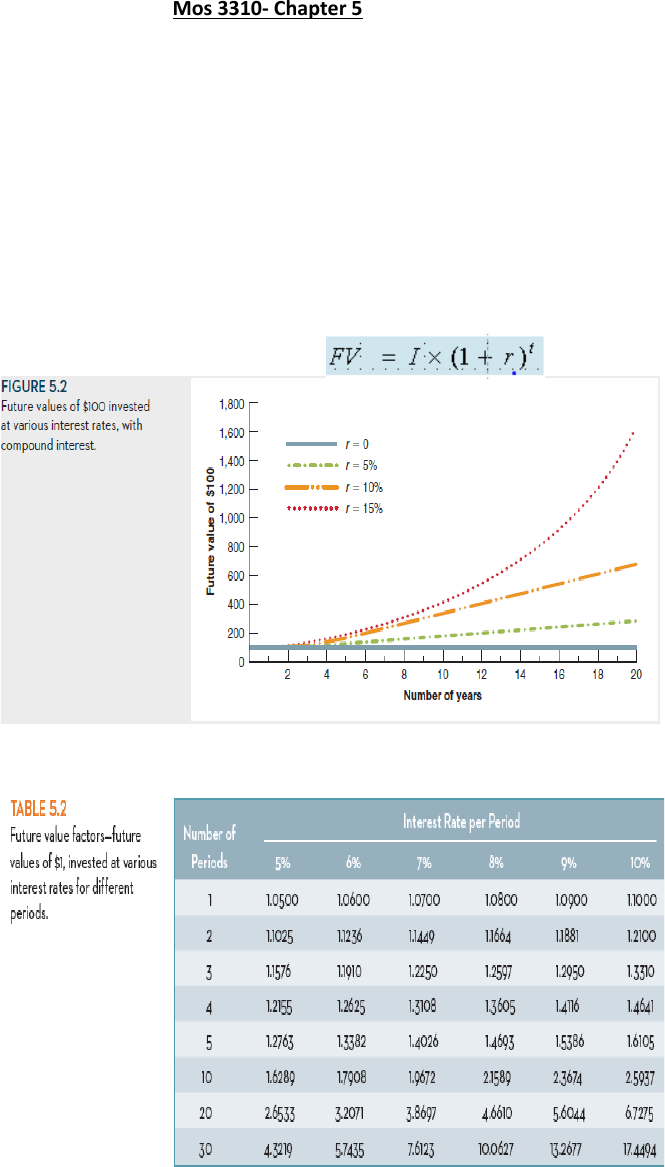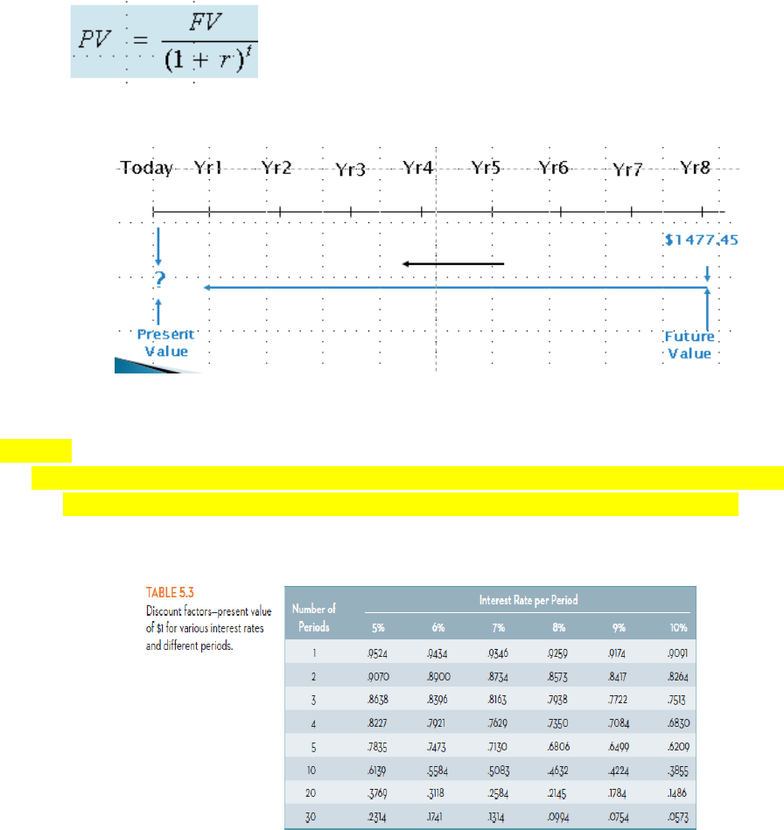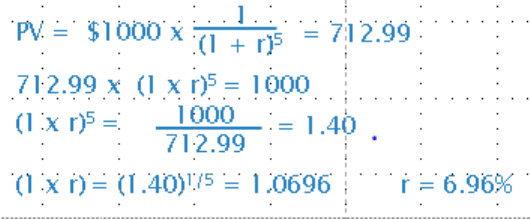Textbook Notes (280,000)
CA (170,000)
Western (10,000)
MOS (2,000)
Chapter 5

# Management and Organizational Studies 3310A Chapter Notes - Chapter 5: Interest, Cash Flow, Nominal Interest Rate

Department
Management and Organizational Studies
Course Code
MOS 3310A
Professor
Bill Dawson
Chapter
5

This preview shows pages 1-2. to view the full 8 pages of the document.Mos 3310- Chapter 5
5.1 Future Values and Compound Interest
Financial decisions require comparisons of cash payments at different dates.
Since a dollar today does not have the same value as a dollar tomorrow, a relationship has to be
established to compare cash flows at different times.
Future value is the amount to which a present value will grow after earning interest.
There are two kinds of interest that money can earn:
o Simple interest- Interest only earned on original investment
o Compound interest-Interest is earned on the value of money that is in the account at
the beginning of the period. Thus, previous period’s earned interest can also earn
interest on the next period. Formula:
o
Time Value Tables:

Only pages 1-2 are available for preview. Some parts have been intentionally blurred.5.2 Present Values
Present value is the value today of a future cash flow.
How much do you need to invest today into an account paying compound interest at the rate of
5% per year, in order to receive \$1477.45 at the end of eight years? (ANS: FV= I x (1+r)^t Thus:
PV= 1477.45/(1.05)^8=\$1000)
Formula:
Present Values:
Example:
You have been offered \$1 million five years from now. If the interest rates is expected to be
10% per year, how much is this prize worth to you in today’s dollars? -EXAM Question
ANS: 1000000/1.1^5=\$620921

Unlock to view full version

Only pages 1-2 are available for preview. Some parts have been intentionally blurred.Finding the Interest rate:
Use the tables look up the discount factor
Rearrange the expression
Example:
PV = 712.99; t = 5 year period; FV=1000
What is the discount rate?
Financial Calculator:
The basic financial calculator uses 5 keys that correspond to the inputs for common problems
involving the time value of money.
N = number of periods (t in formula)
i = the interest rate per period, expressed as a percentage (not a decimal, ie. 8 for 8%,
not .08)
PV = present value
FV = future value
PMT = amount of any recurring payment (called an annuity). For single cash-flow
problems (the ones we have done so far), PMT = 0.
Given any 4 of these inputs, the calculator will calculate the 5th.
5.3 Multiple Cash Flows
Future Value of Multiple Cash Flows
Example:
You deposit \$1,200 in your bank account today; \$1,400 one year later; and \$1,000 two years
from today. If your bank offers you an 8% interest rate on your account, how much money will
you have in the account three years from today?